PCI Express

This lecture is based on the Peripheral Component Interconnect Express,
which is a standard for computer expansion cards.

More specifically, this is a standard for the communication link by which a
PCIe device communicates with the CPU.

According to Wikipedia, PCIe 3.0 (August 2007) is the latest standard.

The PCIe standard is an outgrowth of the original PCI standard, but is not
compatible with PCI at the hardware level.

The PCIe standard is based on a new protocol for electrical signaling.
This protocol is built on the concept of a lane, which we must define.

Here are some capacity quotes from Wikipedia

Per Lane              16–Lane Slot
Version 1            250 MB/s            4 GB/s
Version 2            500 MB/s            8 GB/s
Version 3            1 GB/s                16 GB/s

What is a Lane?

A lane is pair of point–to–point serial links.

It is a full–duplex link, able to communicate in two directions simultaneously.
Each of the serial links in the pair handles one of the two directions.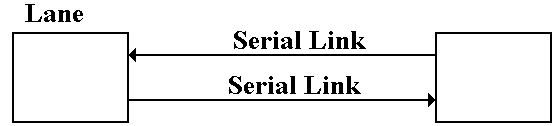By definition, a serial link transmits one bit at a time.

By extension, a lane may transmit two bits at any one time,
one bit in each direction.

One may view a parallel link, transmitting multiple bits in one direction
at any given time, as a collection of serial links.

The only difference is that a parallel link must provide for synchronization
of the bits sent by the individual links.

Data Transmission Codes

The PCIe standard is byte oriented, in that it should be viewed logically
as a full–duplex byte stream.  What is actually transmitted?

The association of bits (transmitted or received) with bytes is handled at the
Data Link layer.  Suppose a byte is to be transmitted serially.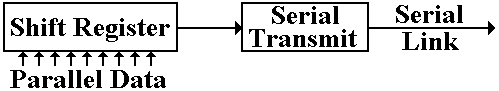The conversion from byte data to bit–oriented data for serial transmission
is done by a shift register.  The register takes in eight bits at a time and
shifts out one bit at a time.

The bits, as shifted out, are still represented in standard logic levels.

The serial transmit unit takes the standard logic levels as input, and
converts them to voltage levels appropriate for serial transmission.

Three Possible Transmission Codes

The serial transmit unit sends data by asserting a voltage on the serial link.

On simple method would be as follows.
To transmit a logic 1, assert +5 volts on the transmission line.
To transmit a logic 0, assert 0 volts on the transmission line.

This simple method has so many difficulties in practice that it cannot be used.
Two of the most obvious are transmission of power and lack of data framing.

Standard methods for link management use codes that avoid these problems.
Two of the more common methods used are NRZ and NRZI.

Non–Return–to–Zero coding transmits by asserting the following voltages:
For a logic 1, it asserts a positive voltage (3.0 – 5.0 volts) on the link.
For a logic 0, it asserts a negative voltage (–3.0 to –5.0 volts).

Non–Return–to–Zero–Invert is a modification of NRZ, using the same
voltage levels.

The Problem of Noise

One problem with these serial links is that they function as antennas.
They will pick up any stray electromagnetic radiation if in the radio range.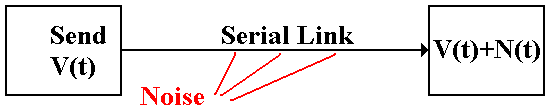In other words, the signal received at the destination might not be what
was actually transmitted.  It might be the original signal, corrupted by noise.

The solution to the problem of noise is based on the observation that two links
placed in close proximity will receive noise signals that are almost identical.

To make use of this observation, we use differential transmitters to send the
signals and differential receivers to reconstruct the signals.

In differential transmission, rather than asserting a voltage on a single output
line, the transmitter asserts two voltages: +V/2 and –V/2.

A +6 volt signal would be asserted as two: +3 volts and –3 volts.

A –8 volt signal would be asserted as two: –4 volts and +4 volts.

Here is a standard diagram of a differential transmitter.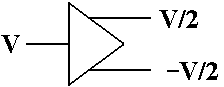The standard differential receiver is an analog subtractor.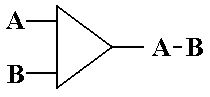For a 6 volt transmitted signal, we have A = 3, B = –3; A – B = 3 – (–3) = 6.

We now assume that the lines used to transmit the differential signals are
physically close together, so that each line is subject to the same noise signal.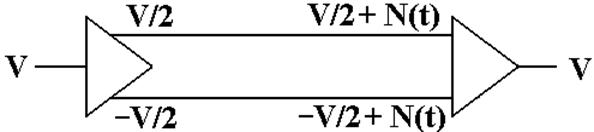Here the received signal is the difference of the two voltages input to

The value received is V/2 – (–V/2) = V, the desired value.

All voltages are measured relative to a standard value, called “ground”.

Here is the complete version of the simple circuit that we want to implement.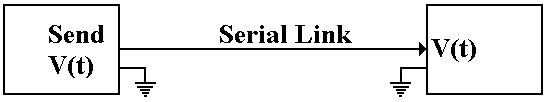Basically, there is an assumed second connection between the two
devices.  This second connection fixes the zero level for the voltage.

There is no necessity for the two devices to have the same ground.  Suppose
that the ground for the receiver is offset from the ground of the transmitter.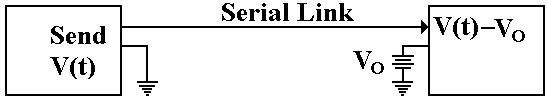The signal sent out as +V(t) will be received as V(t) – VO.

Here again, the subtractor in the differential receiver handles this problem.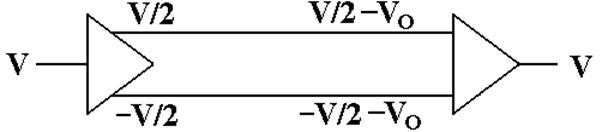The signal originates as a given voltage, which can be positive, negative, or 0.

The signal is transmitted as the pair (+V/2, –V/2).

Due to the ground offset for the receiver, the signal is taken in as
(+V/2 – VO, –V/2 – VO).  The signal is interpreted as
(+V/2 – VO) – (–V/2 – VO) = +V/2 – VO + V/2 + VO = V.

The differential link will correct for both ground offset and line noise
at the same time.

References

1.    Wikipedia    http://en.wikipedia.org/wiki/PCI_Express

2.    Wikipedia       http://en.wikipedia.org/wiki/File:Differential_Signaling.png

3.    Web Site       http://www.interfacebus.com/Design_Connector_RS422.html

4.    Wikipedia    http://en.wikipedia.org/wiki/RS-422